# Persistent Structures in the Code 357 Cellular Automaton

A collection of the persistent structures in the k=3, r=1 totalistic code 357 cellular automaton

These structures were given on page 286 of Stephen Wolfram's "A New Kind of Science"

(4 columns, 6 rows)

## Examples

### Basic Examples

Retrieve the resource:

 In:=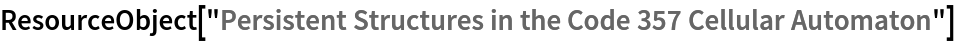Out=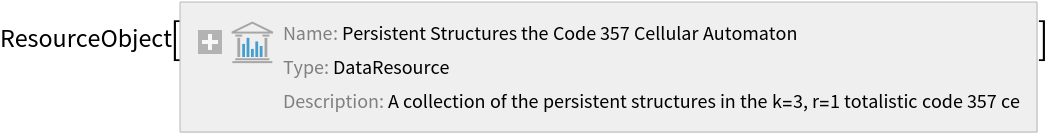Retrieve the default content:

 In:=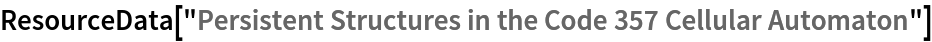Out=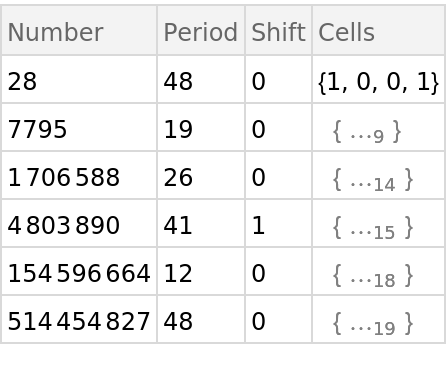Plot the first 200 steps in evolution for these structures:

 In:=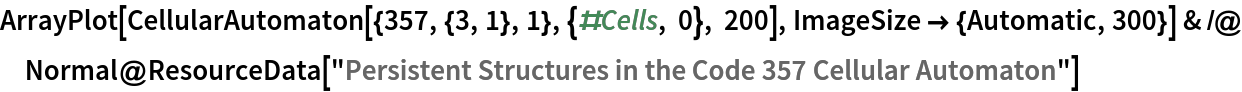Out=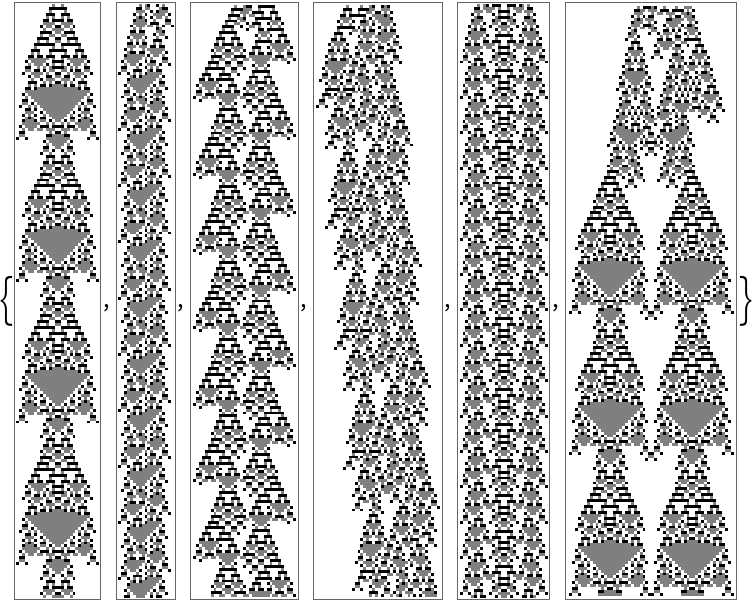Find the repeating element of the third structure:

 In:=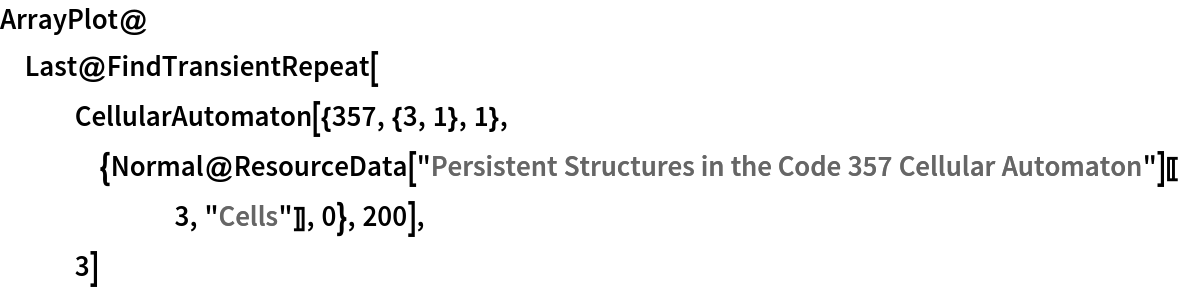Out=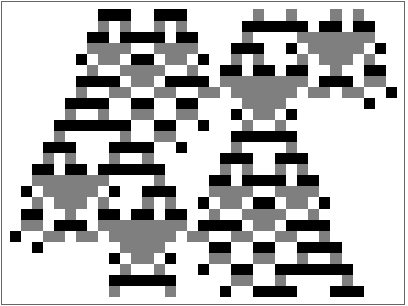In:=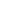Wolfram Research, "Persistent Structures in the Code 357 Cellular Automaton" from the Wolfram Data Repository (2017)